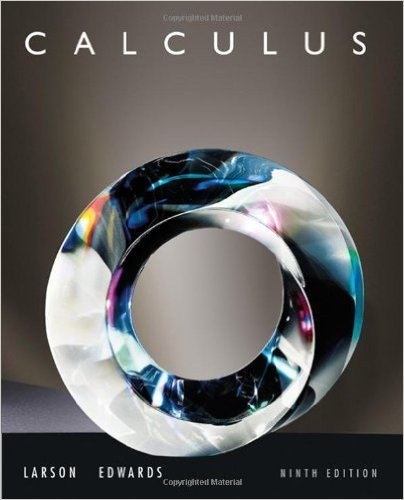×
×

# Solutions for Chapter PS: Multiple Integration## Full solutions for Calculus | 9th Edition

ISBN: 9780547167022Solutions for Chapter PS: Multiple Integration

Solutions for Chapter PS
4 5 0 252 Reviews
19
2
##### ISBN: 9780547167022

Since 18 problems in chapter PS: Multiple Integration have been answered, more than 67796 students have viewed full step-by-step solutions from this chapter. This expansive textbook survival guide covers the following chapters and their solutions. This textbook survival guide was created for the textbook: Calculus , edition: 9. Calculus was written by and is associated to the ISBN: 9780547167022. Chapter PS: Multiple Integration includes 18 full step-by-step solutions.

Key Calculus Terms and definitions covered in this textbook
• Absolute value of a vector

See Magnitude of a vector.

If u < v , then u + w < v + w

• Additive identity for the complex numbers

0 + 0i is the complex number zero

• Arc length formula

The length of an arc in a circle of radius r intercepted by a central angle of u radians is s = r u.

• Combinatorics

A branch of mathematics related to determining the number of elements of a set or the number of ways objects can be arranged or combined

• Common logarithm

A logarithm with base 10.

• Definite integral

The definite integral of the function ƒ over [a,b] is Lbaƒ(x) dx = limn: q ani=1 ƒ(xi) ¢x provided the limit of the Riemann sums exists

• Directed line segment

See Arrow.

• Finite sequence

A function whose domain is the first n positive integers for some fixed integer n.

• Inverse reflection principle

If the graph of a relation is reflected across the line y = x , the graph of the inverse relation results.

• Median (of a data set)

The middle number (or the mean of the two middle numbers) if the data are listed in order.

• Multiplication principle of counting

A principle used to find the number of ways an event can occur.

• Natural numbers

The numbers 1, 2, 3, . . . ,.

• Nonsingular matrix

A square matrix with nonzero determinant

• Projection of u onto v

The vector projv u = au # vƒvƒb2v

• Quotient identities

tan ?= sin ?cos ?and cot ?= cos ? sin ?

• Standard form of a complex number

a + bi, where a and b are real numbers

• Stemplot (or stem-and-leaf plot)

An arrangement of a numerical data set into a specific tabular format.

• Transitive property

If a = b and b = c , then a = c. Similar properties hold for the inequality symbols <, >, ?, ?.

• yz-plane

The points (0, y, z) in Cartesian space.

×